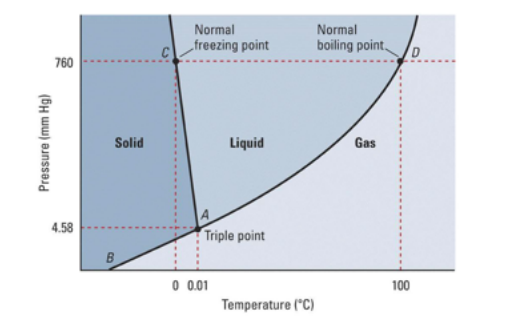Chemistry Practice Problems Phase Diagram Practice Problems Solution: What does letter D represent?  a. fusion curve b...

🤓 Based on our data, we think this question is relevant for Professor Richter-Egger's class at UN OMAHA.

# Solution: What does letter D represent?  a. fusion curve b. triple point c. sublimation point d. melting point e. critical point

###### Problem

What does letter D represent?

a. fusion curve

b. triple point

c. sublimation point

d. melting point

e. critical pointPhase Diagram

Phase Diagram

#### Q. Sulfur can exist as a gas, a liquid, or as one of two solid states: rhombic and monoclinic. A phase diagram for sulfur is shown below: a. What is (ar...

Solved • Wed Dec 14 2016 09:28:34 GMT-0500 (EST)

Phase Diagram

#### Q. A sample is at the temperature and pressure indicated by point (c) on the phase diagram below. What phase is present after the pressure is increased t...

Solved • Mon Sep 19 2016 14:09:57 GMT-0400 (EDT)

Phase Diagram

#### Q. Consider the phase diagram below.  If the dashed line at 1 atm of pressure is followed from 100 to 500°C, what phase changes will occur (in order of i...

Solved • Tue Sep 13 2016 14:57:00 GMT-0400 (EDT)

Phase Diagram

#### Q. Consider the phase diagram shown.  Choose the statement below that is TRUE.   A) The triple point of this substance occurs at a temperature of 31°C. ...

Solved • Tue Sep 13 2016 14:51:38 GMT-0400 (EDT)